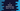# C program to find all disarium numbers from 1 to 100## C program to find all disarium numbers from 1 to 100:

In this post, we will learn how to write a C program that will print all Disarium numbers from 1 to 100. The program we will write will use a loop to find all disarium numbers in between 1 to 100.

With this post, you will learn how to use different types of loops, how to check if a number is disarium number or not and how to do basic mathematical calculations.

Before we move to the final program, let’s understand what is a disarium number and how to check if a number is disarium number or not.

### What is a Disarium number:

A number is called a disarium number if the sum of the digits of the number to the power of its position is equal to the number itself. The position of the digits of a number starts from the leftmost position i.e. the position of the leftmost digit is 1, the second leftmost digit is 2 etc.

Let me show you an example.

### Example of Disarium number:

175 is a disarium number. The position of 1 is 1, position of 7 is 2 and 5 is 3.The sum of the digits of the number each raised to its position is:

``````1^1 + 7^2 + 5^3
= 1 + 49 + 125
= 175``````

That is the number itself. So, it is a disarium number.

### Algorithm to find all disarium numbers from 1 to 100:

The program will use the below algorithm to find all disarium numbers from 1 to 100:

• Run a loop from 1 to 100.
• For each number, check if it is a disarium number or not. If yes, print the number.
• To check if a number is a disarium number or not, create a different function.

• It will take a number as the parameter and return 1 if the number is a disarium number, else it will return 0.
• It will create a copy of the given number.
• Using a loop, it will find the length of the number.
• Using another loop and another copy of the original number, it will find the sum of digits of the number raised to the position of the digit.
• If the sum is equal to the number, it will return 1. Else, it will return 0.

### C program using while loops:

Below is the complete C program:

``````#include <stdio.h>
#include <math.h>

int findLength(int n)
{
int length = 0;
while (n != 0)
{
n /= 10;
length++;
}
return length;
}

int isDisarium(int n)
{
int length = findLength(n);
int copyNo = n;
int lastDigit, sum = 0;

while (copyNo != 0)
{
lastDigit = (int)copyNo % 10;
sum += pow(lastDigit, length);
length--;
copyNo /= 10;
}

return sum == n;
}

int main()
{
int i = 1;

printf("Disarium numbers within 1 to 100 are: ");

while (i <= 100)
{
if (isDisarium(i))
{
printf("%d ", i);
}
i++;
}
printf("\n");

return 0;
}``````
• This program uses only while loops to find all disarium numbers between 1 to 100.
• The main method runs first.

• Inside this method, it uses a while loop that runs for i = 1 to i = 100.
• For each value of i, it calls isDisarium method to check if the value is a disarium number or not.
• If yes, it prints the value of i.
• After each iteration is completed, it increments the value of i by 1.
• The isDisarium method checks if a number is a disarium number or not. It takes a number as the parameter and returns 1 if it is a disarium number. Else, it returns 0.

• It calls findLength to find the length of the number.
• It creates a copy of n and stores it in copyNo.
• By using a while loop, find the last digit of the number, find the power of that digit raised to its position and add it to the sum variable.
• Return 1 if the sum is equal to the number. Else, return 0.
• The findLength method finds the length of a number.

• It uses a while loop to remove the last digit of the number on each step. It increments the value of a length variable which is initialized as 0.
• The while loop stops if the number become 0.
• once the loop ends, it returns the calculated length.

### Output:

If you run this program, it will print all disarium numbers between 1 to 100.

``Disarium numbers within 1 to 100 are: 1 2 3 4 5 6 7 8 9 89 ``

You can change the limit to any number n to find all disarium numbers from 1 to n.

### Method 2: By using a for loop:

We can also use a for loop to write the above program as well. The below program uses for loops:

``````#include <stdio.h>
#include <math.h>

int findLength(int n)
{
int length = 0;
for (; n != 0; n /= 10)
{
length++;
}
return length;
}

int isDisarium(int n)
{
int length = findLength(n);
int lastDigit, sum = 0;

for (int i = n; i != 0; i = i / 10, length--)
{
lastDigit = (int)i % 10;
sum += pow(lastDigit, length);
}

return sum == n;
}

int main()
{
printf("Disarium numbers within 1 to 100 are: ");

for (int i = 1; i <= 100; i++)
{
if (isDisarium(i))
{
printf("%d ", i);
}
}
printf("\n");

return 0;
}``````

If you run this program, it will print the same output.

``Disarium numbers within 1 to 100 are: 1 2 3 4 5 6 7 8 9 89 ``